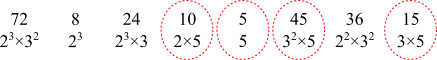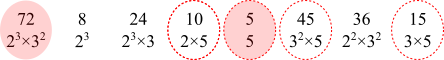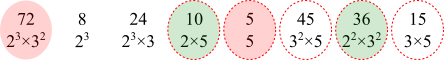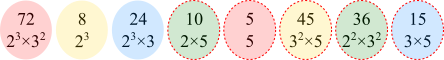#### You may also likeMake a set of numbers that use all the digits from 1 to 9, once and once only. Add them up. The result is divisible by 9. Add each of the digits in the new number. What is their sum? Now try some other possibilities for yourself!### Double Digit### Repeaters

Choose any 3 digits and make a 6 digit number by repeating the 3 digits in the same order (e.g. 594594). Explain why whatever digits you choose the number will always be divisible by 7, 11 and 13.

# Pairing Up

##### Age 11 to 14 ShortChallenge Level

Answer: $36$

Using logic
As the product of each pair has the same value, this value must be product of the smallest and largest numbers, that is $5\times72$.

So the number which is paired with $10$ is $5\times72\div10$, that is $36$.

Using prime factors
Only four have a factor of $5$ so each pair must contain one of these$5$ has no factors of $2$ or $3$, so pairs with $72$, which has the highest powers of $2$ and $3$To get $2^3$ and $3^2$, $10$ pairs with $36$The others can also be paired up:This problem is taken from the UKMT Mathematical Challenges.
You can find more short problems, arranged by curriculum topic, in our short problems collection.